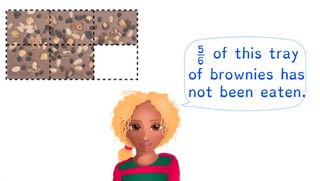Recognizing and writing fractions

# Recognizing and writing fractions

Students learn to recognize and write fractions.

No account needed.8,000 schools use Gynzy92,000 teachers use Gynzy1,600,000 students use Gynzy

## General

The students learn how to recognize and write fractions.

## Standards

CCSS.Math.Content.3.NF.A.1

## Learning objective

Students will be able to recognize and write fractions.

## Introduction

Show a few examples. The students must state which of the images show one and a half and which show three quarters. Drag the images to the correct sides. After this there is a shape on the interactive whiteboard, for which the students must divide the shape into the given number of equal parts. Draw this on the board.

## Instruction

Explain that a fraction consists of a numerator and a denominator, and how you should say a fraction. After this you explain through the image how you can recognize a fraction. First you count how many parts the shape is divided into. Then you count the number of parts that are colored in. If you know these numbers, you can write the fraction. In some cases there are whole figures that are colored in. For these, you write the whole number in front of the fraction. Then you count how many equal parts there are in the incomplete figure and how many are colored in. This makes up the fraction. After this, show a number of images. The fractions are given. The students must choose which image matches the fraction. Next they must come up with what fraction fits each image. Explain that you know which fraction it is by looking at the image. Have the students listen to what the fraction sounds like and show how it then looks when you write it down. The top number of the fraction says how many parts are colored in. The bottom number of the fraction says how many parts the shape is broken up into. Next the students must determine which speaker matches which written fraction. Circle them with matching colors.

To check whether the students can recognize and write fractions, you can ask the following questions:
- How do you write this fraction that you hear: 9//10?
- How do you say this fraction: 1 3//5?

## Quiz

The students test their understanding of recognizing and writing fractions through ten exercises. In some of the exercises they must determine which written fraction matches an image or spoken fraction, and for others they must determine which image or spoken fraction matches a written fraction.

## Closing

You discuss once again with the students that it is useful to be able to recognize and write fractions, so that you know what fraction it is. Check if the students know how to say a fraction out loud. Next you show a number of statements. The students must determine whether each statement is true or false.

## Teaching tips

Students that have difficulty with recognizing and writing fractions can first practice dividing a shape into equal parts. Next they can color in a number of those parts. The next step is recognizing and writing the fraction that they drew. Emphasize that the top number of the fraction tells how many parts are colored in. The bottom number tells how many parts are in the shape.

### The online teaching platform for interactive whiteboards and displays in schools

• Save time building lessons

• Manage the classroom more efficiently

• Increase student engagement